# 51单片机定时器中断的使用

### 基础介绍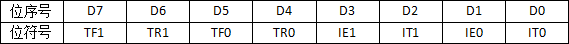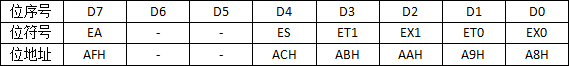ES是串行口中断的开关，ES=1打开串行口中断，ES=0关闭串行口中断。

ET1、EX1、ET0、EX0分别是定时器/计数器1、外部中断1、定时器/计数器0、外部中断0的中断开关，为1时打开相应的中断，为0时关闭相应的中断。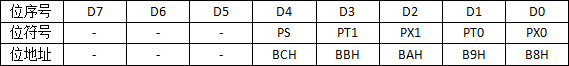1. 打开中断总开关EA；
2. 设置中断优先级（可省略）；
3. 打开对应中断的开关。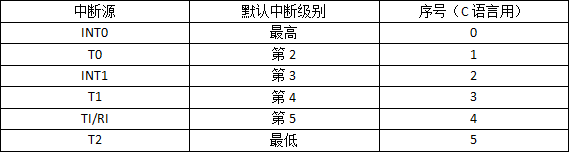### 具体使用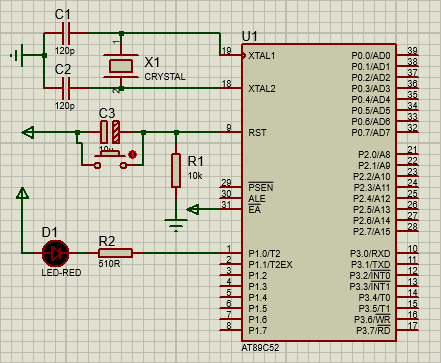```#include <reg51.h>
sbit LED=P1^0;
int a=0;
void main()
{
TMOD=0x01;//使用定时器T0，高4位全为0,低4位GATE=0，C/T=0，采用工作方式1，M1=0,M1=1
TH0=0x3c;//装入初值
TL0=0xae;
TR0=1;//启动定时器0
EA=1;//打开中断总开关
ET0=1;//打开定时器0中断开关
while(1);
}
void abc() interrupt 1
{
TH0=0x3c;//重新装入初值
TL0=0xae;
if(a==20)//因为要1秒闪烁一次，到这里计数器计了0.05s，需要20个0.05s才有1s
{
LED=~LED;
a=0;
}else{
a=++a;
}
}```